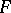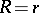# Bonnesen inequality

(diff) ← Older revision | Latest revision (diff) | Newer revision → (diff)
One of the more precise forms of the isoperimetric inequality for convex domains in the plane. Letbe a convex domain in the plane, letbe the radius of the largest circle which can be inserted in, letbe the radius of the smallest circle containing, letbe the perimeter and letbe the area of. The Bonnesen inequalityis then valid. The equalityis attained only if, i.e. ifis a disc. For generalizations of the Bonnesen inequality see .